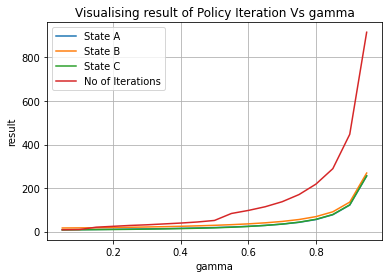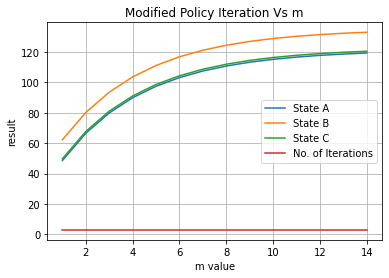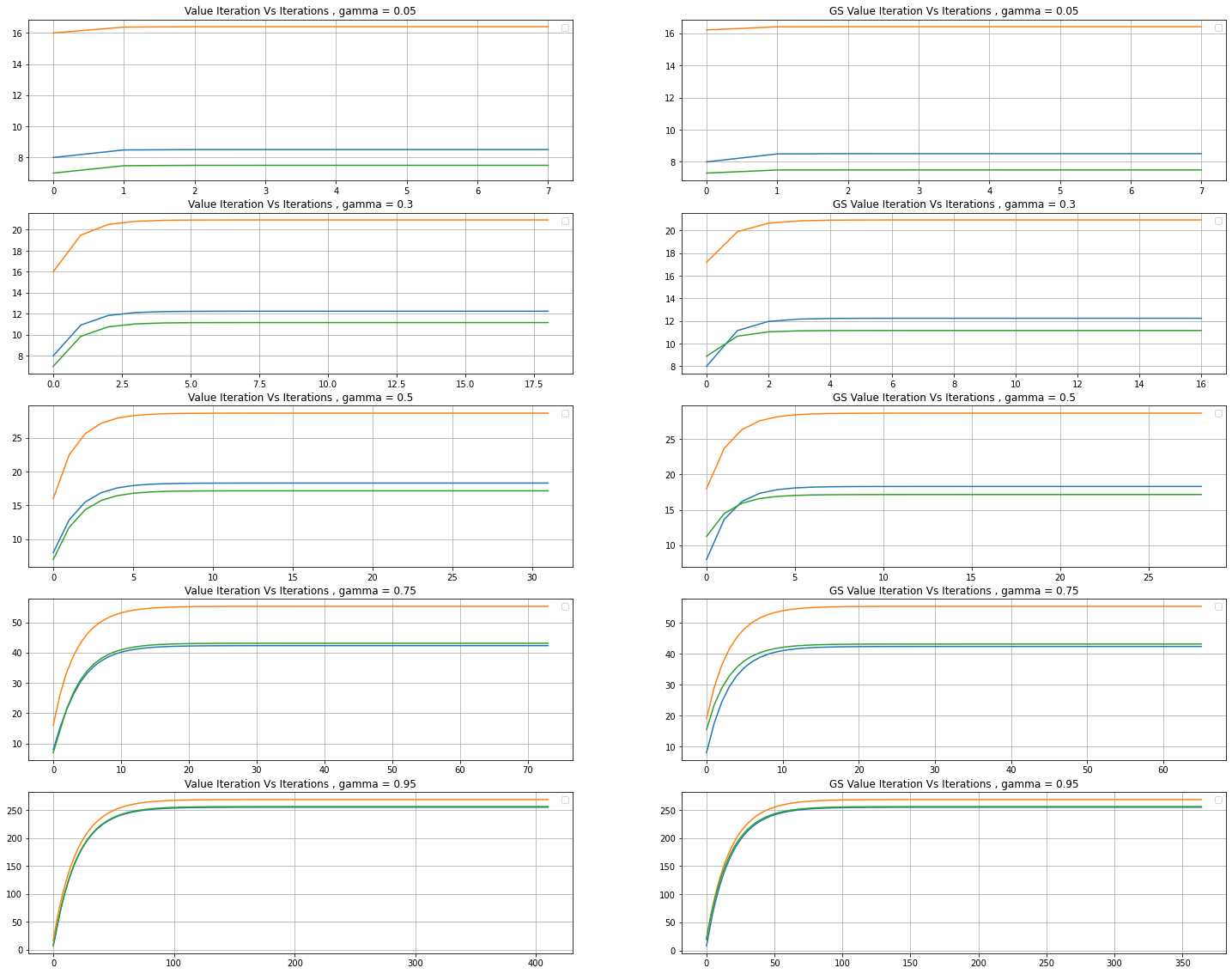# Solution for submission 132192

A detailed solution for submission 132192 submitted for challenge IIT-M RL-ASSIGNMENT-2-TAXI

# What is the notebook about?¶

## Problem - Taxi Environment Algorithms¶

This problem deals with a taxi environment and stochastic actions. The tasks you have to do are:

• Implement Policy Iteration
• Implement Modified Policy Iteration
• Implement Value Iteration
• Implement Gauss Seidel Value Iteration
• Visualize the results
• Explain the results

## How to use this notebook? 📝¶

• This is a shared template and any edits you make here will not be saved.You should make a copy in your own drive. Click the "File" menu (top-left), then "Save a Copy in Drive". You will be working in your copy however you like.

• Update the config parameters. You can define the common variables here

Variable Description
AICROWD_DATASET_PATH Path to the file containing test data. This should be an absolute path.
AICROWD_RESULTS_DIR Path to write the output to.
AICROWD_ASSETS_DIR In case your notebook needs additional files (like model weights, etc.,), you can add them to a directory and specify the path to the directory here (please specify relative path). The contents of this directory will be sent to AIcrowd for evaluation.
AICROWD_API_KEY In order to submit your code to AIcrowd, you need to provide your account's API key. This key is available at https://www.aicrowd.com/participants/me

# Setup AIcrowd Utilities 🛠¶

We use this to bundle the files for submission and create a submission on AIcrowd. Do not edit this block.

In :
!pip install aicrowd-cli > /dev/null

ERROR: google-colab 1.0.0 has requirement requests~=2.23.0, but you'll have requests 2.25.1 which is incompatible.
ERROR: datascience 0.10.6 has requirement folium==0.2.1, but you'll have folium 0.8.3 which is incompatible.


# AIcrowd Runtime Configuration 🧷¶

Get login API key from https://www.aicrowd.com/participants/me

In :
import os

AICROWD_DATASET_PATH = os.getenv("DATASET_PATH", os.getcwd()+"/13d77bb0-b325-4e95-a03b-833eb6694acd_a2_taxi_inputs.zip")
AICROWD_RESULTS_DIR = os.getenv("OUTPUTS_DIR", "results")

In :


API Key valid
Saved API Key successfully!
13d77bb0-b325-4e95-a03b-833eb6694acd_a2_taxi_inputs.zip: 100% 31.2k/31.2k [00:00<00:00, 340kB/s]

In :
!unzip $AICROWD_DATASET_PATH  Archive: /content/13d77bb0-b325-4e95-a03b-833eb6694acd_a2_taxi_inputs.zip creating: inputs/ inflating: inputs/inputs_base.npy inflating: inputs/inputs_1.npy inflating: inputs/inputs_0.npy inflating: inputs/inputs_2.npy creating: targets/ inflating: targets/targets_2.npy inflating: targets/targets_0.npy inflating: targets/targets_1.npy inflating: targets/targets_base.npy  In : DATASET_DIR = 'inputs/'  ## Taxi Environment¶ Read the environment to understand the functions, but do not edit anything In : import numpy as np class TaxiEnv_HW2: def __init__(self, states, actions, probabilities, rewards, initial_policy): self.possible_states = states self._possible_actions = {st: ac for st, ac in zip(states, actions)} self._ride_probabilities = {st: pr for st, pr in zip(states, probabilities)} self._ride_rewards = {st: rw for st, rw in zip(states, rewards)} self.initial_policy = initial_policy self._verify() def _check_state(self, state): assert state in self.possible_states, "State %s is not a valid state" % state def _verify(self): """ Verify that data conditions are met: Number of actions matches shape of next state and actions Every probability distribution adds up to 1 """ ns = len(self.possible_states) for state in self.possible_states: ac = self._possible_actions[state] na = len(ac) rp = self._ride_probabilities[state] assert np.all(rp.shape == (na, ns)), "Probabilities shape mismatch" rr = self._ride_rewards[state] assert np.all(rr.shape == (na, ns)), "Rewards shape mismatch" assert np.allclose(rp.sum(axis=1), 1), "Probabilities don't add up to 1" def possible_actions(self, state): """ Return all possible actions from a given state """ self._check_state(state) return self._possible_actions[state] def ride_probabilities(self, state, action): """ Returns all possible ride probabilities from a state for a given action For every action a list with the returned with values in the same order as self.possible_states """ actions = self.possible_actions(state) ac_idx = actions.index(action) return self._ride_probabilities[state][ac_idx] def ride_rewards(self, state, action): actions = self.possible_actions(state) ac_idx = actions.index(action) return self._ride_rewards[state][ac_idx]  ## Example of Environment usage¶ In : def check_taxienv(): # These are the values as used in the pdf, but they may be changed during submission, so do not hardcode anything states = ['A', 'B', 'C'] actions = [['1','2','3'], ['1','2'], ['1','2','3']] probs = [np.array([[1/2, 1/4, 1/4], [1/16, 3/4, 3/16], [1/4, 1/8, 5/8]]), np.array([[1/2, 0, 1/2], [1/16, 7/8, 1/16]]), np.array([[1/4, 1/4, 1/2], [1/8, 3/4, 1/8], [3/4, 1/16, 3/16]]),] rewards = [np.array([[10, 4, 8], [ 8, 2, 4], [ 4, 6, 4]]), np.array([[14, 0, 18], [ 8, 16, 8]]), np.array([[10, 2, 8], [6, 4, 2], [4, 0, 8]]),] initial_policy = {'A': '1', 'B': '1', 'C': '1'} env = TaxiEnv_HW2(states, actions, probs, rewards, initial_policy) print("All possible states", env.possible_states) print("All possible actions from state B", env.possible_actions('B')) print("Ride probabilities from state A with action 2", env.ride_probabilities('A', '2')) print("Ride rewards from state C with action 3", env.ride_rewards('C', '3')) base_kwargs = {"states": states, "actions": actions, "probabilities": probs, "rewards": rewards, "initial_policy": initial_policy} return base_kwargs base_kwargs = check_taxienv() env = TaxiEnv_HW2(**base_kwargs)  All possible states ['A', 'B', 'C'] All possible actions from state B ['1', '2'] Ride probabilities from state A with action 2 [0.0625 0.75 0.1875] Ride rewards from state C with action 3 [4 0 8]  ## Task 1 - Policy Iteration¶ Run policy iteration on the environment and generate the policy and expected reward In : # 1.1 Policy Iteration def policy_iteration(taxienv, gamma): # A list of all the states states = taxienv.possible_states # Initial values values = {s: 0 for s in states} # This is a dictionary of states to policies -> e.g {'A': '1', 'B': '2', 'C': '1'} policy = taxienv.initial_policy.copy() ## Begin code here # Hints - # Do not hardcode anything # Only the final result is required for the results # Put any extra data in "extra_info" dictonary for any plots etc # Use the helper functions taxienv.ride_rewards, taxienv.ride_probabilities, taxienv.possible_actions # For terminating condition use the condition exactly mentioned in the pdf # Put your extra information needed for plots etc in this dictionary extra_info = {} ####My Code Start...................#Done done = 0 epsilon = 1e-8 NIterations = 0 while(1): while(1): delta = 0 for state_i in states: j = values[state_i] action = policy[state_i] probabs = taxienv.ride_probabilities(state_i,action) rewards = taxienv.ride_rewards(state_i,action) value_tmp = 0 for enm,state_j in enumerate(states): value_tmp+= probabs[enm]*(rewards[enm]+ gamma*values[state_j]) values[state_i] = value_tmp delta = max(delta, abs(j-values[state_i])) NIterations+=1 if(delta<epsilon): break done= 1 for state_i in states: b = policy[state_i] tmp_arr = [] for action in taxienv.possible_actions(state_i): probabs = taxienv.ride_probabilities(state_i,action) rewards = taxienv.ride_rewards(state_i,action) tmp = 0 for enm,state_j in enumerate(states): tmp+= probabs[enm]*(rewards[enm]+ gamma*values[state_j]) tmp_arr.append(tmp) policy[state_i]= taxienv.possible_actions(state_i)[np.argmax(tmp_arr)] if(b != policy[state_i]): done=0 if(done==1): break extra_info["NIterations"] = NIterations ####My Code End..................... ## Do not edit below this line # Final results return {"Expected Reward": values, "Policy": policy}, extra_info  # Task 2 - Policy Iteration for multiple values of gamma¶ Ideally this code should run as is In : # 1.2 Policy Iteration with different values of gamma def run_policy_iteration(env): gamma_values = np.arange(5, 100, 5)/100 results, extra_info = {}, {} for gamma in gamma_values: results[gamma], extra_info[gamma] = policy_iteration(env, gamma) return results, extra_info results, extra_info = run_policy_iteration(env)  # Task 3 - Modifed Policy Iteration¶ Implement modified policy iteration (where Value iteration is done for fixed m number of steps) In : # 1.3 Modified Policy Iteration def modified_policy_iteration(taxienv, gamma, m): # A list of all the states states = taxienv.possible_states # Initial values values = {s: 0 for s in states} # This is a dictionary of states to policies -> e.g {'A': '1', 'B': '2', 'C': '1'} policy = taxienv.initial_policy.copy() ## Begin code here # Hints - # Do not hardcode anything # Only the final result is required for the results # Put any extra data in "extra_info" dictonary for any plots etc # Use the helper functions taxienv.ride_rewards, taxienv.ride_probabilities, taxienv.possible_actions # For terminating condition use the condition exactly mentioned in the pdf # Put your extra information needed for plots etc in this dictionary extra_info = {} ####My Code Start...................#DONE done= 0 NIterations = 0 while(1): for k in range(m+1): H = {s: 0 for s in states} for state_i in states: action = policy[state_i] probabs = taxienv.ride_probabilities(state_i,action) rewards = taxienv.ride_rewards(state_i,action) for enm,state_j in enumerate(states): H[state_i]+= probabs[enm]*(rewards[enm]+ gamma*values[state_j]) for state_i in states: values[state_i] = H[state_i] done = 1 for state_i in states: b = policy[state_i] tmp_arr = [] for action in taxienv.possible_actions(state_i): probabs = taxienv.ride_probabilities(state_i,action) rewards = taxienv.ride_rewards(state_i,action) tmp = 0 for enm,state_j in enumerate(states): tmp+= probabs[enm]*(rewards[enm]+ gamma*values[state_j]) tmp_arr.append(tmp) policy[state_i]= taxienv.possible_actions(state_i)[np.argmax(tmp_arr)] if(b != policy[state_i]): done=0 NIterations+=1 if(done==1): break extra_info["NIterations"] = NIterations ####My Code End..................... ## Do not edit below this line # Final results return {"Expected Reward": values, "Policy": policy}, extra_info  # Task 4 Modified policy iteration for multiple values of m¶ Ideally this code should run as is In : def run_modified_policy_iteration(env): m_values = np.arange(1, 15) gamma = 0.9 results, extra_info = {}, {} for m in m_values: results[m], extra_info[m] = modified_policy_iteration(env, gamma, m) return results, extra_info results, extra_info = run_modified_policy_iteration(env)  # Task 5 Value Iteration¶ Implement value iteration and find the policy and expected rewards In : # 1.4 Value Iteration def value_iteration(taxienv, gamma): # A list of all the states states = taxienv.possible_states # Initial values values = {s: 0 for s in states} # This is a dictionary of states to policies -> e.g {'A': '1', 'B': '2', 'C': '1'} policy = taxienv.initial_policy.copy() ## Begin code here # Hints - # Do not hardcode anything # Only the final result is required for the results # Put any extra data in "extra_info" dictonary for any plots etc # Use the helper functions taxienv.ride_rewards, taxienv.ride_probabilities, taxienv.possible_actions # For terminating condition use the condition exactly mentioned in the pdf # Put your extra information needed for plots etc in this dictionary extra_info = {} ####My Code Start...................#DONE Converged = False epsilon = 1e-8 NIterations = 0 extra_info["ValuesEvolution"] = [] #Declare H H = {} for state in states: H[state] = None while(not Converged): delta = 0 for state_i in states: H[state_i] = 0 tmp_arr= [] for action in taxienv.possible_actions(state_i): H_comp= 0 probabs = taxienv.ride_probabilities(state_i,action) rewards = taxienv.ride_rewards(state_i,action) for enm, state_j in enumerate(states): H_comp+= probabs[enm]*(rewards[enm]+ gamma*values[state_j]) tmp_arr.append(H_comp) H[state_i] = np.max(tmp_arr) policy[state_i] = taxienv.possible_actions(state_i)[np.argmax(tmp_arr)] delta = max(delta,abs(values[state_i]-H[state_i])) for state_i in states: values[state_i]=H[state_i] NIterations+=1 extra_info["ValuesEvolution"].append(values.copy()) if(delta<epsilon): Converged = True extra_info["NIterations"] = NIterations ####My Code End..................... ## Do not edit below this line # Final results return {"Expected Reward": values, "Policy": policy}, extra_info  # Task 6 Value Iteration with multiple values of gamma¶ Ideally this code should run as is In : def run_value_iteration(env): gamma_values = np.arange(5, 100, 5)/100 results = {} results, extra_info = {}, {} for gamma in gamma_values: results[gamma], extra_info[gamma] = value_iteration(env, gamma) return results, extra_info results, extra_info = run_value_iteration(env)  # Task 7 Gauss Seidel Value Iteration¶ Implement Gauss Seidel Value Iteration In : # 1.4 Gauss Seidel Value Iteration def gauss_seidel_value_iteration(taxienv, gamma): # A list of all the states # For Gauss Seidel Value Iteration - iterate through the values in the same order states = taxienv.possible_states # Initial values values = {s: 0 for s in states} # This is a dictionary of states to policies -> e.g {'A': '1', 'B': '2', 'C': '1'} policy = taxienv.initial_policy.copy() # Hints - # Do not hardcode anything # For Gauss Seidel Value Iteration - iterate through the values in the same order as taxienv.possible_states # Only the final result is required for the results # Put any extra data in "extra_info" dictonary for any plots etc # Use the helper functions taxienv.ride_rewards, taxienv.ride_probabilities, taxienv.possible_actions # For terminating condition use the condition exactly mentioned in the pdf ## Begin code here # Put your extra information needed for plots etc in this dictionary extra_info = {} ####My Code Start...................#DONE Converged = False epsilon = 1e-8 NIterations=0 extra_info["ValuesEvolution"] = [] while(not Converged): delta = 0 for state_i in states: j = values[state_i] tmp_arr = [] for action in taxienv.possible_actions(state_i): probabs = taxienv.ride_probabilities(state_i, action) rewards = taxienv.ride_rewards(state_i, action) tmp = 0 for enm, state_j in enumerate(states): tmp+= probabs[enm]*(rewards[enm]+ gamma*values[state_j]) tmp_arr.append(tmp) values[state_i]= np.max(tmp_arr) policy[state_i]= taxienv.possible_actions(state_i)[np.argmax(tmp_arr)] delta = max(delta, abs(j-values[state_i])) NIterations+=1 extra_info["ValuesEvolution"].append(values.copy()) if(delta<epsilon): Converged = True extra_info["NIterations"] = NIterations ####My Code End................... ## Do not edit below this line # Final results return {"Expected Reward": values, "Policy": policy}, extra_info  # Task 8 Gauss Seidel Value Iteration with multiple values of gamma¶ Ideally this code should run as is In : def run_gauss_seidel_value_iteration(env): gamma_values = np.arange(5, 100, 5)/100 results = {} results, extra_info = {}, {} for gamma in gamma_values: results[gamma], extra_info[gamma] = gauss_seidel_value_iteration(env, gamma) return results, extra_info results, extra_info = run_gauss_seidel_value_iteration(env)  # Generate Results ✅¶ In : # Do not edit this cell def get_results(kwargs): taxienv = TaxiEnv_HW2(**kwargs) policy_iteration_results = run_policy_iteration(taxienv) modified_policy_iteration_results = run_modified_policy_iteration(taxienv) value_iteration_results = run_value_iteration(taxienv) gs_vi_results = run_gauss_seidel_value_iteration(taxienv) final_results = {} final_results["policy_iteration"] = policy_iteration_results final_results["modifed_policy_iteration"] = modified_policy_iteration_results final_results["value_iteration"] = value_iteration_results final_results["gauss_seidel_iteration"] = gs_vi_results return final_results  In : # Do not edit this cell, generate results with it as is if not os.path.exists(AICROWD_RESULTS_DIR): os.mkdir(AICROWD_RESULTS_DIR) for params_file in os.listdir(DATASET_DIR): kwargs = np.load(os.path.join(DATASET_DIR, params_file), allow_pickle=True).item() results = get_results(kwargs) idx = params_file.split('_')[-1][:-4] np.save(os.path.join(AICROWD_RESULTS_DIR, 'results_' + idx), results)  # Check your local score¶ This score is not your final score, and it doesn't use the marks weightages. This is only for your reference of how arrays are matched and with what tolerance. In : # Check your score on the given test cases (There are more private test cases not provided) target_folder = 'targets' result_folder = AICROWD_RESULTS_DIR def check_algo_match(results, targets): param_matches = [] for k in results: param_results = results[k] param_targets = targets[k] policy_match = param_results['Policy'] == param_targets['Policy'] rv = [v for k, v in param_results['Expected Reward'].items()] tv = [v for k, v in param_targets['Expected Reward'].items()] rewards_match = np.allclose(rv, tv, rtol=3) equal = rewards_match and policy_match param_matches.append(equal) return np.mean(param_matches) def check_score(target_folder, result_folder): match = [] for out_file in os.listdir(result_folder): res_file = os.path.join(result_folder, out_file) results = np.load(res_file, allow_pickle=True).item() idx = out_file.split('_')[-1][:-4] # Extract the file number target_file = os.path.join(target_folder, f"targets_{idx}.npy") targets = np.load(target_file, allow_pickle=True).item() algo_match = [] for k in targets: algo_results = results[k] algo_targets = targets[k] algo_match.append(check_algo_match(algo_results, algo_targets)) match.append(np.mean(algo_match)) return np.mean(match) if os.path.exists(target_folder): print("Shared data Score (normalized to 1):", check_score(target_folder, result_folder))  Shared data Score (normalized to 1): 1.0  ## Visualize results of Policy Iteration with multiple values of gamma¶ Add code to visualize the results In : ## Visualize policy iteration with multiple values of gamma import matplotlib.pyplot as plt results_POL_Iter_gamma, extra_info_POL_Iter_gamma = run_policy_iteration(env) vizDict = {} states = env.possible_states gammas = sorted(results_POL_Iter_gamma.keys()) for state in states: vizDict[state] = [] vizDict["NIterations"] = [] for gamma in gammas: vizDict["NIterations"].append(extra_info_POL_Iter_gamma[gamma]["NIterations"]) for state in states: vizDict[state].append(results_POL_Iter_gamma[gamma]["Expected Reward"][state]) for state in states: plt.plot(gammas, vizDict[state]) plt.plot(gammas, vizDict["NIterations"]) plt.xlabel("gamma") plt.ylabel("result") plt.title("Visualising result of Policy Iteration Vs gamma") plt.grid(True) plt.legend(list(map(lambda x: "State "+ x, states))+["No of Iterations"]) plt.show()# Subjective questions¶ ## 1.a How are values of$\gamma$affecting results of policy iteration¶ Modify this cell/add code for your answer. From the above plot we can see that as the gamma value increases the Final converged value or in case the result of Policy Iteration is also found to increase along with the increase in the number of iterations inorder to converge to that final value or result. From the graph we can also see a characteristic exponential increase in the final converged value with increase in gamma. (This is expected as discount factor >1 should cause it to diverge and go to infinity and this is convinced as we can extrapolate the graph and can realise it tends to touch a asymptote at gamma = 1). ## 1.b For modified policy itetaration, do you find any improvement if you choose m=10.¶ Explain your answer From the below graph with the increase in m, we can see that the final converged result increased (Actually tends towards the Policy iterations results with large m's) but this happens without the increase in the number of iterations (It is found to be constant of 3). As observed from graph it seems to asymtotically approach a upper value and that value is actually Policy iteration's convergence result which is the one expected and this happens without even increasing the number of iterations which is actually an improvement as we are getting a consistent result there after even with higher m values. In : results_ModPOL_Iter_mValues, extra_info_ModPOL_Iter_mValues = run_modified_policy_iteration(env) states = env.possible_states mVals = sorted(results_ModPOL_Iter_mValues.keys()) vizDict = {} for state in states: vizDict[state] = [] vizDict["mVals"]= [] for mVal in mVals: NIterations = extra_info_ModPOL_Iter_mValues[mVal]["NIterations"] vizDict["mVals"].append(NIterations) for state in states: vizDict[state].append(results_ModPOL_Iter_mValues[mVal]["Expected Reward"][state]) plt.figure(1) plt.xlabel("m value") plt.ylabel("result") plt.title("Modified Policy Iteration Vs m") for state in states: plt.plot(mVals, vizDict[state]) plt.plot(mVals, vizDict["mVals"]) plt.legend(list(map(lambda x: "State "+ x, states))+ ["No. of Iterations"]) plt.grid(True) plt.show()## 1.c Compare and contrast the behavior of Value Iteration and Gauss Seidel Value Iteraton¶ Add code/markdown as required From the plots below we can see that irrespective of gamma both Value Iteration and Gauss Seidel Value Iteration are converging to the same final reward but they differ in the number of Iterations taken by them to converge. Gauss Seidel Value Iteration is found to converge faster than the Value Iteration. The difference is found to scale up with gamma and as gamma increases the Iterations needed to converge also increases consequently causing the difference in convergence between Value and Gauss Seidel Value Iteration is also found to increase. In : results_ValIter, extra_info_ValIter = run_value_iteration(env) results_GS_ValIter, extra_info_GS_ValIter = run_gauss_seidel_value_iteration(env) states = env.possible_states gammas = sorted(results_ValIter.keys()) gammas = np.array(gammas).take([0,5, 9 ,-5,-1]) #ValueIter vizDict1 = {} for gamma in gammas: vizDict1[gamma] = {} for state in states: vizDict1[gamma][state] = [] for gamma in gammas: #Write data for state in states: for ele in extra_info_ValIter[gamma]["ValuesEvolution"]: vizDict1[gamma][state].append(ele[state]) #GaussSeidelValueIter vizDict2 = {} for gamma in gammas: vizDict2[gamma] = {} for state in states: vizDict2[gamma][state] = [] for gamma in gammas: #Write data for state in states: for ele in extra_info_GS_ValIter[gamma]["ValuesEvolution"]: vizDict2[gamma][state].append(ele[state]) #Plot fig, a = plt.subplots(len(gammas),2, figsize = (25,20)) #aset(xlabel = "Iterations", ylabel = "results") for enm,gamma in enumerate(gammas): for state in states: a[enm].plot(vizDict1[gamma][state]) a[enm].grid(True) a[enm].legend(map(lambda x: "State "+ x, states)) a[enm].plot(vizDict2[gamma][state]) a[enm].grid(True) a[enm].legend(map(lambda x: "State "+ x, states)) a[enm].set_title("Value Iteration Vs Iterations , gamma = "+str(round(gamma,2))) a[enm].set_title("GS Value Iteration Vs Iterations , gamma = "+str(round(gamma,2))) plt.show()# Submit to AIcrowd 🚀¶ In [ ]: !DATASET_PATH=$AICROWD_DATASET_PATH aicrowd notebook submit -c iit-m-rl-assignment-2-taxi -a assets

WARNING: No assets directory at assets... Creating one...
No jupyter lab module found. Using jupyter notebook.
Using notebook: /content/AE17B030_IITM_Assignment_2_Taxi_Release.ipynb for submission...